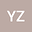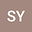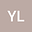The special least squares solutions of the complex matrix equation (AXB,CXD)=(E,F)
•••• yanzhen Zhang,
• Shanshan Yang,
• Ying Li
yanzhen Zhang
Liao Cheng University

Corresponding Author:1065920035@qq.com

Author ProfileShanshan Yang
Beijing Institute of Technology
Author ProfileYing Li
Liao Cheng University
Author Profile## Abstract

In this paper, we study the problem of least square solutions of the complex matrix equation (AXB,CXD)=(E,F). First, by using the real representation method of the complex matrix, we prosent the real representation form of the complex matrix equation (AXB,CXD)=(E,F). In combination with the special structure of the real representation matrix of the complex matrix, the vec operator of the matrix, the Kronecker product of the matrix and the MP inverse property of the matrix, we obtain the Hermitian least squares solution of the complex matrix equation(AXB,CXD)=(E,F),and derive the Hermitian minimum norm least square solution, the real symmetric minimum norm least square solution and the real dissymmetric minimum norm least square solution of the complex matrix equation(AXB,CXD)=(E,F). At last, we give the expressions of three minimum norm least squares solutions and their corresponding algorithms, respectively.